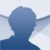# Microsoft Math Solver Android

1.0.183

## Microsoft Math Solver is a calculator developed by Microsoft for solving mathematical problems in areas such as algebra, trigonometry or statistics

Among other devices that have made smartphones obsolete are calculators... especially with applications like Microsoft Math Solver. The app developed by the American company is capable of solving operations in different areas of mathematics and also explains what the process has been.

### A perfect application for students

This is an excellent application to solve mathematical operations of calculation, arithmetic, algebra, trigonometry, statistics... it has a solver equipped with its own AI that is capable of interpreting any type of operation, either written by the user himself or registered through the camera of the smartphone.

But it doesn't only solve problems: it offers a whole series of resources, including video, which explains the process of solving the problem, making it an ideal tool for anyone who wants to know how to carry out one of these operations.

These are the main features:

• Write mathematical equations on the screen as you would on paper.
• Scan photos or handwritten math problems.
• Interactive solutions explained step by step.
• Graphing calculator.
• Scan and solve full sheets of math problems.
• Search for similar math problems on the web.
• Solve linear and non-linear functions.
• Provides solutions and explanations in several languages.